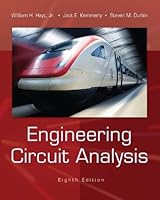# Engineering Circuit Analysis, 8th Edition## Book Description

The hallmark feature of this classic text is its focus on the student - it is written so that students may teach the science of to themselves. Terms are clearly defined when they are introduced, basic material appears toward the beginning of each chapter and is explained carefully and in detail, and numerical examples are used to introduce and suggest general results. Simple practice problems appear throughout each chapter, while more difficult problems appear at the end of chapters, following the order of presentation of text material. This introduction and resulting repetition provide an important boost to the learning process.

Hayt's rich pedagogy supports and encourages the student throughout by offering tips and warnings, using to highlight key material, and providing lots of opportunities for hands-on learning. The thorough exposition of topics is delivered in an informal way that underscores the authors' conviction that analysis can and should be fun.

Chapter 1 Introduction
Chapter 2 Basic Components And Electric Circuits
Chapter 3 Voltage And Current Laws
Chapter 4 Basic Nodal And Mesh Analysis
Chapter 5 Handy Circuit Analysis Techniques
Chapter 6 The Operational Amplifier
Chapter 7 Capacitors And Inductors
Chapter 8 Basic Rl And Rc Circuits
Chapter 9 The Rlc Circuit
Chapter 11 Ac Circuit Power Analysis
Chapter 12 Polyphase Circuits
Chapter 13 Magnetically Coupled Circuits
Chapter 14 Complex Frequency And The Laplace Transform
Chapter 15 Circuit Analysis In The S-Domain
Chapter 16 Frequency Response
Chapter 17 Two-Port
Chapter 18 Fourier Circuit Analysis

Appendix 1 An Introduction To Topology
Appendix 2 Solution Of Simultaneous Equations
Appendix 3 A Proof Of Thévenin’S Theorem
Appendix 4 A Pspice® Tutorial
Appendix 5 Complex Numbers
Appendix 6 A Brief ® Tutorial
Appendix 7 Additional Laplace Transform Theorems